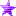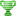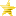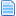# Sin (math), Cos (math), and Tan (math) (Triggers) (Read 1477 times)

Started by Ricepigeon, September 17, 2015, 10:39:05 pm

#### Ricepigeon##### Sin (math), Cos (math), and Tan (math) (Triggers)
#1  September 17, 2015, 10:39:05 pm
••• Gaps? Where we're going, we don't need gaps...
Computes the sine, cosine, or tangent of the specified argument (in radians.)

Format:
1) sin(exprn)
2) cos(exprn)
3) tan(exprn)
Arguments:
exprn
Expression to compute the sine, cosine, or tangent of. (float)
Return type:
float
Error conditions:
Returns bottom if exprn evaluates to bottom.

Examples:
Code:
`value = sin(pi/2)  Sets value to the sine of pi/2, which is approximately 1.0  (possibly with some rounding error.)value = cos(0)  Sets value to the cosine of 0, which is approximately 1.0  (possibly with some rounding error.)value = tan(pi/4)  Sets value to the tangent of pi/4, which is approximately 1.0 (possibly with some rounding error.)`

Note that the Sin, Cos, and Tan triggers only accept radian values as arguments. In order to compute the Sin, Cos, or Tan of a value in degrees, the value must first be converted into radian form by multiplying by Pi and dividing by 180, for example;

Code:
`value = sin(90*Pi/180.0)`

This will set value to the Sin of 90 degrees, which is approximately 1.0. The expression of 90*Pi/180 is the conversion of the value of the angle from degree to radian form.

Another thing to note is that because the tangent of 90 degrees is mathematically undefined, attempting to compute that value using Tan will return Bottom. This also holds true for other values where the tangent of that value is undefined (i.e., 270 degrees, 450 degrees, 630 degrees, etc).
Last Edit: September 18, 2015, 02:47:07 pm by Just No Point

#### vgma2##### Re: Sin (math), Cos (math), and Tan (math) (Triggers)
#2  September 20, 2015, 04:26:31 am
••• Lost in a sea of Smash
Honestly, I'm surprised this wasn't posted before arcsin, arccos, and arctan.